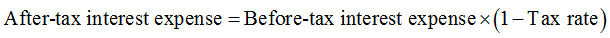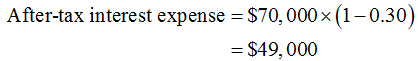# A firm incurs \$70,000 in interest expenses each year. If the tax rate of the firm is 30%, what is the effective after-tax interest rate expense for the firm?

Question
1 views

A firm incurs \$70,000 in interest expenses each year. If the tax rate of the firm is 30%, what is the effective after-tax interest rate expense for the firm?

check_circle

Step 1

The below expression can be used to calculate after-tax interest expense:Step 2

After-tax interest expense is calculated below:...

### Want to see the full answer?

See Solution

#### Want to see this answer and more?

Solutions are written by subject experts who are available 24/7. Questions are typically answered within 1 hour.*

See Solution
*Response times may vary by subject and question.
Tagged in